# Information rates of precoding for massive MIMO and base station cooperation in an indoor scenario

## Abstract

The performance of centralized and distributed massive MIMO deployments are studied for simulated indoor office scenarios. The distributed deployments use one of the following precoding methods: (1) local precoding with local channel state information (CSI) to the user equipments (UEs) that it serves, (2) large-scale MIMO with local CSI to all UEs in the network, (3) network MIMO with global CSI. For the distributed deployment (3), it is found that using twice as many base station antennas as data streams provides many of the massive MIMO benefits in terms of spectral efficiency and fairness. This is in contrast to the centralized and distributed deployments using (1) or (2) where more antennas are needed. Two main conclusions are that distributing base stations helps to overcome wall penetration loss; however, a backhaul is required to mitigate inter-cell interference. The effect of estimation errors on the performance is also quantified.

## Introduction

One goal of new mobile radio communication standards, e.g., 5th generation mobile networks (5G) , is to increase the spectral efficiency (SE) per unit area or volume. For example, the METIS (Mobile and wireless communications Enablers for the Twenty-twenty Information Society) project  defines target traffic volume densities for different scenarios. One way to increase SE is by using multiple-input multiple-output (MIMO) schemes. MIMO allows one node to transmit several streams to one or more user equipments (UEs) using spatial degrees of freedom.

Massive MIMO refers to a “vast” over-provisioning of base station (BS) antennas as compared to the number of served single antenna UEs . Massive MIMO is also known as “Very Large MIMO,” “Hyper MIMO,” “Full Dimension MIMO,” “Large-Scale Antenna Systems,” or “ARGOS” . However, the term massive MIMO is not clearly defined. Massive MIMO may refer to any MIMO configuration beyond the largest MIMO mode in the current LTE standard (at present 8x8), e.g., 100 antennas or more , or it may simply refer to a “large“ number of antennas at the BSs. A more precise definition of massive MIMO is based on the ratio M/K of serving BS antennas M to the number K of active UEs. However, the ratio M/K for which one can speak of massive MIMO depends on the performance metric, the scenario, etc. .

Massive MIMO claims several advantages over conventional MIMO :

• Massive MIMO increases capacity by 10 times or more and simultaneously increases energy efficiency. The transmit signals are directed precisely to the UEs through precoding which reduces interference. Each additional antenna increases the precoding degrees of freedom assuming no mutual coupling and a sufficiently complex propagation environment [5, 7].

• Inexpensive, low-power components suffice. A large number of BS antennas makes the system robust against noise, fading, and hardware impairments or even failure of antenna elements. This allows simpler transmitters and receivers at the BS, e.g., few or one bit quantization, hybrid digital-analog precoding, and constant envelope precoding .

• Precoding simplifies. Simple linear precoding has a vanishing gap to optimal precoding [3, 5, 6]. For instance, the performance gap between linear zeroforcing beamforming (ZFBF)  and the optimal, non-linear dirty paper coding (DPC)  vanishes with an increasing number of BS antennas. Maximum ratio transmission (MRT) is also asymptotically optimal as the number of BS antennas increases, but for a smaller number of BS antennas, MRT performs well only in the low signal-to-noise ratio (SNR) regime .

• The multiple-access layer simplifies. The channel hardens by the law of large numbers [4, 5]. This means that all subcarriers experience similar small-scale fading and the UE channel vectors become orthogonal. Hence, scheduling does not improve performance because all UEs can be active on all subcarriers. Only power control is needed to distribute the power depending on the slowly varying large-scale fading .

• The latency is reduced. Since all UEs can always be active, UEs need not wait for good fading conditions.

• Massive MIMO is robust to jamming and interference. The surplus of precoding degrees of freedom can be used to cancel interference or jamming.

Most massive MIMO studies consider wide area outdoor scenarios [3, 4, 6]. However, most mobile traffic is generated by indoor users . We study the downlink performance of different BS deployments with different levels of cooperation for the 3rd Generation Partnership Project (3GPP) indoor office scenario  and discuss the following questions. What ratio M/K is required to achieve the massive MIMO advantages? How large is the gain of distributing antennas and of cooperation? How does cooperation involving outdoor BSs perform? How much is performance affected by channel estimation error?

Our approach is as follows. We fix the number of active, single antenna UEs and sweep the ratio M/K from one to ten. The BSs use suboptimal transmission schemes and we study fairness using Jain’s index . Placing a single massive MIMO BS at the center of a building causes the UEs to experience large path loss and high wall penetration loss. Also, it was found that distributed MIMO increases diversity . We compare a centralized single BS deployment to distributed BSs with three levels of cooperation: local precoding with local channel state information (CSI) to the UEs that it serves, large-scale MIMO (LS-MIMO) with local CSI to all active UEs, and network MIMO with global CSI. Like in conventional MIMO, CSI is required to enable precoding. We assume perfect CSI for the first parts of the study and then quantify the performance loss due to channel estimation error.

We find that a ratio of twice as many BS antennas as served UEs is a good trade-off between number of antennas versus SE. For network MIMO, this ratio provides most of the massive MIMO benefits while more antennas are needed with local precoding, LS-MIMO, or the single BS deployment. The performance of the suboptimal transmission schemes approaches a capacity upper bound. Fairness increases with the number of BS antennas, with the level of cooperation between BSs, and with the distribution of BS antennas (given some cooperation level between the BSs). We find that distributed indoor BSs with cooperation achieve a substantial performance gain at the cost of a backhaul connection, similar to , while the gain achieved with cooperation between outdoor BSs and a single indoor BS is smaller. With increasing capability of the backhaul, the cooperation level can be increased which allows to achieve the same performance with fewer BS antennas. Good channel estimation is crucial to obtain the benefits of massive MIMO and especially the benefits of network MIMO.

Our results should help to guide the design of future mobile radio communication systems, e.g., Long-Term Evolution-Advanced (LTE-Advanced) and 5G. We presented preliminary results in , and we add the following aspects.

• Instead of using water-filling to allocate power, we use mercury/water-filling, which is optimal for finite modulation alphabets .

• We study two additional deployments (the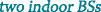deployment and the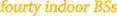deployment).

• We add LS-MIMO as an example of an interference coordination scheme.

• We study fairness for Gaussian modulation.

A version of this work containing further details especially on the preliminaries is available online .

Bold lowercase letters denote vectors, and bold uppercase letters denote matrices. The transpose of X is XT and the complex conjugate transpose is XH. The Euclidean norm of x is x2, and the Frobenius norm of X is XF. A diagonal matrix having diagonal entries x is denoted by diag(x).

## Methods

### System model

Consider the downlink in the 3GPP “A1 - Indoor Office” scenario in the Wireless World Initiative New Radio II (WINNER II) deliverable , see Fig. 1. The UEs are served by BSs located inside and outside the building. The K single antenna UEs use orthogonal frequency-division multiplexing (OFDM). For each subcarrier, one obtains a broadcast channel (BC). The received signal of the k-th UE on the f-th subcarrier is

$$y_{k}^{{(f)}} = \left(\mathbf{h}_{k}^{{(f)}}\right)^{\mathsf{H}} \mathbf{x}^{{(f)}} + z_{k}^{{(f)}}, \quad k\in\left\{1,\ldots,K\right\}$$
(1)

where $$\mathbf {h}_{k}^{\mathsf {H}} = \left [\mathbf {h}_{k,1}^{\mathsf {H}}, \ldots, \mathbf {h}_{k,N_{\text {BS}}}^{\mathsf {H}}\right ]$$ is the vector of channel coefficients from all NBS BSs to the k-th UE. The subcarrier index is omitted if f is clear from the context. The i-th BS has Mi BS antennas with the channel coefficients $$\mathbf {h}_{k,i}^{\mathsf {H}}$$. The dimension of $$\mathbf {h}_{k}^{\mathsf {H}}$$ is $$M=\sum _{i=1}^{N_{\text {BS}}} M_{i}$$. The transmit signal vectors are collected in $$\mathbf {x} = \left [\mathbf {x}_{1}^{\mathsf {T}}, \ldots, \mathbf {x}_{N_{\text {BS}}}^{\mathsf {T}} \right ]^{\mathsf {T}}$$, and the z1,z2,…,zK are independent proper complex additive white Gaussian noise (AWGN) random variables with variance $$\sigma _{N}^{2}$$. The received signals y=[y1,…,yK]T of all UEs for one subcarrier are collected in the vector

$$\mathbf{y} = \mathbf{{H}}^{\mathsf{H}} \mathbf{x} + \mathbf{z}$$
(2)

where $$\mathbf {{H}}^{\mathsf {H}} = \left [\mathbf {h}_{1}^{\mathsf {H}},\ldots,\mathbf {h}_{K}^{\mathsf {H}}\right ]$$ and z=[z1,…,zK]T.

For linear precoding, the transmit signals vector x is

$$\mathbf{x} = \mathbf{{W}} \mathbf{s}$$
(3)

where W=[w1,…,wK] is the matrix of the precoding vectors and s=[s1,…,sK]T is the vector of transmit symbols. Let $$\mathbb {E}\left [\left |s_{k}\right |^{2}\right ]=1$$ for k{1,2,…,K}, and consider the per-BS sum-power constraints

$$\sum_{f=1}^{N_{\text{SC}}} \mathbb{E}\left[\left\|\mathbf{x}_{i}^{(f)}\right\|^{2}_{2}\right] = \sum_{f=1}^{N_{\text{SC}}}\left\|\mathbf{{W}}^{(f)}_{i} \right\|^{2}_{F} \leq P_{i} \quad\forall i \in \left\{1,\ldots, N_{\text{BS}}\right\}$$
(4)

where NSC is the number of subcarriers, and $$\mathbf {{W}}^{(f)}_{i}$$ is the part of the precoding matrix that creates the transmit signals at the ith BS $$\mathbf {x}^{(f)}_{i}$$.

### Transmission schemes

Interference management is important for modern wireless communication standards like Long-Term Evolution (LTE) , LTE-Advanced , and for future standards like 5G. A general framework and optimization algorithms for multi-cell scenarios with different levels of cooperation are presented in . We are interested in transmission schemes with low complexity. For ease of notation, the principle is described for a single subcarrier and the subcarrier index is omitted.

#### Local precoding

Local precoding BSs determine the transmit signals and the scheduled UEs locally. They treat inter-cell interference as noise, and thus interference limits reliable transmission in many scenarios. As a result, backhaul requirements are low and only local CSI is required.

Suppose each UE is served by the BS with the maximum average SNR, and ZFBF  is used to mitigate intra-cell interference. The local precoding matrix at the ith BS is

$$\mathbf{{W}}_{i} = \mathbf{{H}}_{i,i}\left(\mathbf{{H}}_{i,i}^{\mathsf{H}}\mathbf{{H}}_{i,i}\right)^{-1} \text{diag}\left(\tilde{\mathbf{p}}_{i}\right)^{\frac{1}{2}}$$
(5)

where $$\mathbf {{H}}_{i,i}^{\mathsf {H}}$$ is the channel matrix from the ith BS to its Ki UEs, and $$\tilde {\mathbf {p}}_{i}$$ is the power allocation vector at the ith BS. ZFBF requires that the ith BS serves at most Mi UEs, i.e., KiMi. If Ki>Mi then the low complexity scheduling algorithm from  is used to select Mi UEs. Note that the set of scheduled UEs may be different on each subcarrier. Each BS uses mercury/water-filling  to allocate power according to a per-BS power constraint. For ill-conditioned channels, the performance improves when using other precoding strategies, e.g., [9, 26].

#### Large-scale MIMO

Interference coordination has each BS estimate its channels to all UEs and exchange this CSI with the other BSs. The resulting global CSI lets us coordinate the transmissions of the BSs, e.g., by power allocation, precoding, and scheduling. A UE is served by a single BS. The backhaul requirements are modest because a signal-level synchronization of the BSs is not needed . The coordination can be accomplished at a central processor or locally at the BSs. The distributed, local coordination can be realized in a competitive (game theoretic) way or with the help of control messages over the backhaul. Note that to reduce the backhaul requirements some coordination schemes exchange little CSI, and some schemes exchange control messages instead.

The coordination schemes can be categorized as follows :

• Coordinated scheduling (CS) has the scheduling and power allocation optimized jointly by all BSs.

• Coordinated beamforming (CB) has the precoding coordinated using available precoding degrees of freedom to reduce interference.

• The combination of CS and CB, which is called coordinated scheduling/ coordinated beamforming (CS/CB), is more common than pure CB.

• For CS, CB, and CS/CB, interference is treated as noise. Performance can improve if interference is detected at the UEs . Interference detection can be supported by coding at the transmitter, e.g., by interference alignment.

We consider large-scale MIMO (LS-MIMO)  as an example of an interference coordination scheme. LS-MIMO is a linear CB scheme which does not exchange CSI or control messages over the backhaul. However, it requires sufficiently many antennas: the number Mi of antennas at the ith BS must be at least as large as the total number of UEs, i.e., MiK. Then, each BS uses ZFBF to mitigate the interference created at all UEs and thereby creates parallel interference-free channels to the UEs it serves. The UEs served by each BS are determined based on maximal SNR as for local precoding. Note that LS-MIMO is feasible only if MNBSK. It can be made feasible by scheduling a subset of UEs. However, we study LS-MIMO only if MNBSK. Each BS uses ZFBF via

$$\mathbf{{W}}_{i} = \mathbf{{H}}_{i}\left(\mathbf{{H}}_{i}^{\mathsf{H}}\mathbf{{H}}_{i}\right)^{-1} \text{diag}\left(\tilde{\mathbf{p}}_{i}\right)^{\frac{1}{2}}$$
(6)

where $$\mathbf {{H}}_{i}^{\mathsf {H}}$$ is the channel matrix from the ith BS to all UEs, and each BS allocates power by mercury/water-filling . Local precoding approaches the zero-forcing behavior of LS-MIMO with increasing Mi because the channels to the UEs of the other BSs become orthogonal to the channels of the served UEs .

A similar approach is eigen-direction-aware zero forcing, which shows promising results for a similar scenario .

#### Network MIMO

Network MIMO requires that the BSs are connected by a backhaul with low delay and high throughput and that the BSs are synchronized. The distributed BSs act as one BS with distributed antennas, and the downlink channel becomes a BC. In contrast to interference coordination, network MIMO may have interference enhance the signals at the UEs. Network MIMO can be realized by a central processor or by exchanging messages between the BSs.

For the network MIMO scheme, we assume a perfect backhaul with unlimited capacity and zero delay. The rationale for this assumption is that we aim to understand the potential of network MIMO; the design of strategies to deal with an imperfect backhaul are out of the scope of this work. All BSs act as a single BS with distributed antennas and apply ZFBF with per-BS power constraints. The classic multiple-access channel (MAC)-BC duality does not determine the optimal precoder for per-BS power constraints . A low-complexity and suboptimal approach is to determine for each subcarrier the ZFBF precoding matrix

$$\mathbf{{W}} = \mathbf{{H}}\left(\mathbf{{H}}^{\mathsf{H}}\mathbf{{H}}\right)^{-1} \text{diag}\left(\tilde{\mathbf{p}}\right)^{\frac{1}{2}}$$
(7)

where $$\tilde {\mathbf {p}}$$ is the power allocation vector. Mercury/water-filling  serves to allocate power according to a total power constraint

$$\sum_{f=1}^{N_{\text{SC}}}\mathbb{E}\left[\left\|\mathbf{x}^{(f)}\right\|^{2}_{2}\right] \leq \sum_{i=1}^{N_{\text{BS}}} P_{i}.$$
(8)

Next, each BS determines its transmit power and scales its precoding matrix W so that the per-BS power constraint is satisfied at the BS with the maximal transmit power. Note that the other BSs could transmit with higher power. Hence, this is a suboptimal approach, and better approaches can be found, e.g., see [30, 31].

Network MIMO helps to avoid rank deficient and poorly conditioned channel matrices which are caused by spatial correlations or by the “keyhole” effect . Network MIMO is sometimes called “distributed MIMO,” “MIMO cooperation,” “coherently coordinated transmission,” “Joint Processing CoMP,” “Joint Transmission CoMP,” “C-RAN (Cloud-RAN),” or “p-cell” .

## Simulation setup

### Indoor scenario

Figure 1 shows the layout of the indoor office scenario defined as “A1 - Indoor Office” in the WINNER II deliverable D.1.1.2 . The UEs are located 1.5 m above the floor inside the building. The Quasi Deterministic Radio Channel Generator (QuaDRiGa)  is used to generate the channel coefficients.

The indoor channels are generated according to the “A1 - Indoor Office” channel model parameters . There are two parameter sets for line-of-sight (LOS) and for non line-of-sight (NLOS) conditions. For NLOS conditions, a wall penetration loss is added, where the wall penetration loss is determined by counting the number of walls between each BS and UE beyond the first penetrated wall. When counting the number of walls, paths along the corridors are considered as alternatives to the direct path, which might penetrate more walls.

The outdoor-to-indoor channels are generated according to the “B4 - Outdoor to indoor” channel model parameters defined in the Wireless World Initiative New Radio + (WINNER +) deliverable D5.3 . The outdoor BSs are below rooftop micro BSs. We assume a LOS path from the BS to the outside wall of the building. For each UE, the pathloss is calculated based on the path through the point on an outside wall nearest to the UE. The number of penetrated walls is determined as for the indoor BSs.

#### Base station deployments

We define six different BS deployments which are shown in Fig. 1.

• s13638-019-1636-5fmcc.eps[JWFFXGRAPHICS] is a single BS with M antennas located in the corner of the room southwest of the center (“sceOne1” in Fig. 1). This is a classical massive MIMO deployment.

• s13638-019-1636-5fmcd.eps[JWFFXGRAPHICS] are two BSs with M/2 antennas each. One BS is located in the center of each corridor (“sceTwo2”).

• s13638-019-1636-5fmce.eps[JWFFXGRAPHICS] are four BSs with M/4 antennas each. Two BSs are located in each corridor (“sceSeven3”).

• s13638-019-1636-5fmcf.eps[JWFFXGRAPHICS] are fourty BSs with M/40 antennas each. One BS is located in the center of each room (“sceNine4”). This is similar to the deployment of p-cell .

• s13638-019-1636-5fmcg.eps[JWFFXGRAPHICS] are two BSs with M/2 antennas each. They are located 15 m north/south of the middle of the north/south outside wall (“sceTen5”).

• s13638-019-1636-5fmch.eps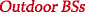deployment (“sceOne1”) while two are in the location of thedeployment (“sceTen5”).

Note that all deployments except the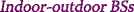deployment require a high-capacity backhaul (not shown in Fig. 1), which might be wireless , to permit network MIMO. Also note that the BSs are not necessarily optimally placed.

#### Antenna array configuration

The indoor BSs are rectangle arrays, while the outdoor BSs are uniform linear arrays (ULAs). The antennas are spaced at half wavelength distance λL/2. The rectangular arrays are mounted underneath the ceiling at a height of 3 m. The side lengths of the rectangle are such that $$\lceil \sqrt {M_{i}} \rceil$$ antennas fit per row and column. Note that the last rows might not be fully occupied. The height of the outdoor BSs is 10 m and the antennas of the ULAs are located on a line parallel to the long side of the building. We assume no mutual coupling between antennas. Unless otherwise stated, we assume ideal hardware, perfect synchronization, and perfect CSI of the complete network at all nodes.

### Simulation parameters

We fix the number of UEs to K=24 and compare the deployments with different performance measures for different numbers M of total BS antennas. Three hundred drops are simulated where one drop is a random placement of the 24 UEs within the office building. For each drop, 10 channel realizations are generated. The wall penetration loss is 12 dB per wall. The bandwidth is 20 MHz around a carrier frequency of 2.1 GHz. The active bandwidth is 18 MHz and 1 MHz on each side of this bandwidth is a guard band. The subcarrier spacing is 15 kHz and one obtains 1200 subcarriers. In LTE, subcarriers are arranged in groups of 12 consecutive subcarriers which are called physical resource blocks (PRBs). Hence, one obtains 100 PRBs. The channel conditions of the subcarriers of one PRB are usually very similar. The schedule, power allocation, and precoder are the same for all subcarriers of one PRB in LTE to save control signaling overhead. We save simulation time by simulating a single subcarrier per PRB and assuming that the same performance is achieved on the other subcarriers of the PRB.

Unless otherwise mentioned, the modulation is 256 quadrature amplitude modulation (QAM) and mercury/water-filling is used to allocate power. The per-BS power in dBm at the ith BS is constrained by

$$P_{i} = {26} \text{dBm} - 10 \log_{10}\left(N_{\text{BS}}\right).$$
(9)

The maximal per-BS powers are such that the maximal sum power available to the BSs is 26 dBm. The variance of the AWGN at the UEs, i.e., the noise level, is $$\sigma _{N}^{2} = {-125.1} \text {dBm}$$.

The simulation parameters are summarized in Table 1. With these parameters, the per-UE SE Sk of the kth UE without considering control signaling overhead is

$$S_{k} = \frac{12 \cdot \sum_{f=1}^{100} C\left(\text{SINR}_{k}^{(f)}\right) \cdot 14 }{ {1} \text{ms} \cdot {20} \text{MHz}}$$
(10)

where 12 is the number of subcarriers per PRB, 100 is the number of PRBs, 14 is the number of OFDM blocks per subframe, 1 ms is the duration of one subframe, and $$C\left (\text {SINR}_{k}^{(f)}\right)$$ is the capacity at $$\text {SINR}_{k}^{(f)}$$ of a memoryless channel with 256 QAM input and continuous output in bits . The sum SE S in the building without considering control signaling overhead is

$$S = \sum_{k=1}^{24} S_{k}$$
(11)

where 24 is the number of UEs. The maximal sum SE for 256 QAM is S=161.28 bit/s/Hz, since the rate $$C\left (\text {SINR}_{k}^{(f)}\right)$$ is bounded by 8 bits for 256 QAM.

## Results and discussion

### Sum spectral efficiency

First, consider the average sum SE S. The 5 percentile sum SE and the 95 percentile sum SE follow the same trends and are not shown. For the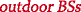deployment, there is only one BS; hence, the curves for local precoding, LS-MIMO, and network MIMO are equal.

Consider the sum SE achieved with network MIMO (solid curves) in Fig. 2. The deployments perform poorly for the fully loaded MIMO system with M=24 BS antennas. The sum SE improves significantly when few antennas are added. Adding more antennas increases the sum SE, but the gain per additional antenna decreases. A ratio of twice as many BS antennas as UEs seems to be a good trade-off between achieved sum SE and number of BS antennas. As expected, the distributed deployments outperform thedeployment, except for the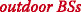deployment which performs poorly with all transmission schemes.

Next, consider the sum SE achieved with LS-MIMO (dashed curves) in Fig. 2. Recall that for LS-MIMO at least M=NBSK total BS antennas are required. Similar to network MIMO, adding more antennas increases the sum SE, and the gain with each additional antenna decreases. Since LS-MIMO does not require a backhaul, one can trade off the costs of a backhaul with the number M of BS antennas to achieve the sum SE of network MIMO with LS-MIMO.

Local precoding is non-cooperative and performs poorly due to interference (dotted curves), see Fig. 3. For all deployments, the sum SE improves little when adding antennas. However, it may be beneficial to distribute BS antennas even without cooperation. For example, the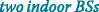deployment with local precoding outperforms the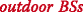deployment. Local precoding outperforms network MIMO for small M when more UEs are served by a BS than the BS can serve with local precoding and only the best UEs are scheduled.

In conclusion, the SE increases with the number of BS antennas for all deployments and all transmission schemes until it is limited by the maximal SE of the modulation. Cooperation between indoor BSs provides large gains, while cooperation between outdoor BSs or indoor and outdoor BSs provides smaller gains. Network MIMO performs best, but CS/CB is an interesting alternative as the backhaul requirements are reduced. The placement of BSs is important to overcome wall penetration losses and to control interference.

A SE of 100 bit/s/Hz without considering overhead is achievable with 192 antennas using local precoding and less than 28 antennas using two indoor BSs with network MIMO. Considering an overhead of 50%, the required bandwidth to achieve the goals of the METIS project  is:

• For the TC1 virtual reality office:

$$\frac{{{0.1\ \text{bit/s/m}}^{2}} \cdot {{5000} \mathrm{m}^{2}}}{{50}\ \text{bit/s/Hz}} = {10}\ \text{GHz}.$$
(12)

More UE antennas, more base stations, or larger QAM constellations could reduce the required bandwidth.

• For the TC2 dense urban information society:

$$\frac{{{0.7}\ \text{Mbit/s/m}^{2}} \cdot {{{5000} m}^{2}}}{{50}\ \text{bit/s/Hz}} = {70}\ \text{MHz}.$$
(13)

This performance is achievable with single antenna UEs, few BSs, and 256 QAM within a reasonable bandwidth.

### Average SNR maps

This subsection provides reasons as to why the deployments with only one or no indoor BS perform poorly as compared to the distributed indoor BSs deployments. To this end, only a single UE is served at different positions within the office building and its average SNR is measured. The BSs use network MIMO under per-BS power constraints,Footnote 1 and they distribute the per-BS transmit power equally among the subcarriers. The SNR achieved when a single UE is served is an upper bound to the SNR when more UEs are served with ZFBF or any other linear precoding scheme, as serving more UEs only reduces the degrees of freedom.

Figure 4 shows the SNRs averaged over 300 channel realizations for each sampled position. The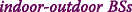deployment achieves low SNR in many rooms, especially those close to the outside wall. This is due to the wall penetration loss. The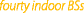deployment and the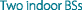deployment achieve low SNR in inner rooms and in the corridors. The other deployments achieve high SNR in all rooms.

We conclude that the lower SEs of the deployments with only one or no indoor BS are at least partly due to the large wall penetration loss and the building penetration loss. A deployment with few well-placed BSs suffices to provide good service throughout the building.

### Comparison to capacity upper bound

Massive MIMO lets simple transmission schemes approach capacity with an increasing number of BS antennas. In the following, this statement is studied for the network MIMO transmission scheme. One can upper bound the capacity of a deployment by the capacity of a BC under a total power constraint. Suppose all BSs of a deployment cooperate and act as one BS with distributed antennas, and suppose we relax the per-BS power constraint to a total-power constraint. Note that for the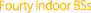deployment, the upper bound is tight, as the capacity of a BC is achieved by non-linear DPC . The algorithms in  find the optimal transmission policy while treating the OFDM subcarriers as virtual antennas. The resulting capacity is compared to the SEs achieved with Gaussian modulation, since 256 QAM limits SE, while Gaussian modulation allows to approach the capacity upper bound.

Figure 5 shows the capacity upper bounds and the average sum SEs achieved with Gaussian modulation and network MIMO under per-BS power constraints and under a total power constraint. The general trends are similar to Figs. 2 and 3, but the SEs increase without bound with the number of BS antennas. For few BS antennas, the gap between the capacity upper bound and the network MIMO rates is large, but the gap could be reduced by more advanced scheduling algorithms. The channels harden for more BS antennas: it becomes optimal to schedule all UEs on each subcarrier , and advanced scheduling strategies provide diminishing gains . With an increasing number of BS antennas, the gap decreases and vanishes completely under a total power constraint, while a gap remains under per-BS power constraints. Determining better capacity upper bounds and choosing better precoding and power allocation under per-BS power constraints would reduce the gap. In summary, network MIMO allows simple transmission schemes to approach capacity with an increasing number of BS antennas in our scenarios.

### Fairness study

The deployments and transmission schemes should provide a fair service to all UEs as the channels harden. One can measure fairness quantitatively with Jain’s index 

$$J \left(S_{1}, S_{2}, \dots, S_{K} \right) = \frac{\left(\sum_{k=1}^{K} S_{k} \right)^{2}}{K \cdot \sum_{k=1}^{K} {S_{k}}^{2}}.$$
(14)

Jain’s index is 1 when all UEs achieve the same per-UE SE and is 1/K when only one UE achieves a positive per-UE SE.

Figure 6 shows the simulated fairness indices. For network MIMO and LS-MIMO, the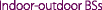deployment, the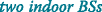deployment, and thedeployment approach perfect fairness indices of 1 with an increasing number of BS antennas. This is partly due to all UEs being served with the maximal per-UE SE of 256 QAM. With Gaussian modulation, the trends of Jain’s fairness index are similar, but no deployment achieves perfect fairness. For local precoding, the fairness indices are lower and they do not approach a fairness index of 1 in the considered range of BS antennas. The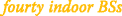deployment, thedeployment, and the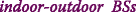deployment do not approach a fairness index of 1 with any transmission scheme in the range of BS antennas, but the index increases with the number M of BS antennas.

We conclude that fairness increases with the number of BS antennas, with the level of cooperation between BSs, and with the distribution of BS antennas (given some cooperation between BSs). Note that one can increase fairness by making it an objective while scheduling and allocating power.

### Noisy channel estimation

So far, we assumed perfect CSI. However, perfect CSI is usually not available, and acquiring CSI might be difficult in massive MIMO due to the many antennas. Frequency division duplex (FDD) requires a pilot sequence for each BS antenna, while time division duplex (TDD) might suffer from pilot contamination . Hence, a study of the effect of estimation errors on the average SE is in order. Let the channel coefficient with estimation error from the mth antenna of the ith BS to the kth UE at subcarrier f be

$$\hat{h}_{i,k,m}^{(f)} = h_{i,k,m}^{(f)} + e_{i,k,m}^{(f)}$$
(15)

where $$h_{i,k,m}^{(f)}$$ is the channel coefficient without error and $$e_{i,k,m}^{(f)}$$ is the estimation error. The estimation errors are independent and zero-mean proper complex Gaussian random variables. The estimation error of the channel between the ith BS and the kth UE is normalized such that its variance scales with the mean channel coefficient squared

$$\mathbb{E}\left[\left|e_{i,k,m}^{(f)} \right|^{2}\right] = \mathbb{E}\left[\left|h_{i,k,m}^{(f)}\right|\right]^{2} \sigma_{E}^{2}$$
(16)

where $$\sigma _{E}^{2}$$ is the normalized mean squared error (NMSE), and the expectation is over the BS antennas and the subcarriers. This channel estimation error occurs, e.g., for channel prediction [15, 43]. The BSs determine the precoders based on the channel estimation with error. For these precoders, intra-cell interference occurs due to the estimation error.

Figure 7 shows the average SEs versus NMSE for 48 total transmit antennas, except for thedeployment where only 40 total BS antennas are deployed. With network MIMO, the performance of all deployments severely degrades with increasing NMSE. The SEs of local precoding are unaffected by low NMSE and degrade for high NMSE only. Inter-cell interference is always present for local precoding and dominates over the interference caused by channel estimation errors for most of the NMSE range. Hence, the power allocation of local precoding is more robust to interference and local precoding outperforms network MIMO for a NMSE higher than −30 to −20 dB. However, the performance of network MIMO with estimation errors can be improved, e.g., by making the power allocation more robust to the additional interference caused by estimation errors . For more BS antennas, the trends and performance differences are similar.

We conclude that all deployments suffer from channel estimation noise, while some deployments are more sensitive. Good channel estimation is crucial to obtain the massive MIMO and network MIMO benefits. However, more robust precoding techniques and power allocation schemes could improve performance in the presence of prediction errors.

## Conclusions

We compared the performance of six different deployments and different levels of cooperation in the 3GPP indoor office scenario. Cooperation between BSs provides gains as compared to no cooperation, which become larger as the level of cooperation increases. The same performance as a single massive MIMO BS is achieved by distributed BSs with cooperation and fewer antennas. The costs of antenna elements can be traded off with the costs for backhaul capability to achieve the same performance. Network MIMO with a ratio of twice as many BS antennas as served UEs can offer many of the massive MIMO benefits. User fairness and SE close to capacity are achieved with simple transmission schemes. Accurate channel estimation is necessary to obtain the massive MIMO and cooperation benefits.

## Availability of data and materials

The datasets used and/or studied during the current study are available from the corresponding author on reasonable request.

1. 1.

For a single served UE, ZFBF coincides with maximum ratio transmission.

## Abbreviations

3GPP:

3rd Generation Partnership Project

5G:

5th generation mobile networks

AWGN:

BC:

BS:

Base station

CB:

Coordinated beamforming

CS:

Coordinated scheduling

CS/CB:

Coordinated scheduling/coordinated beamforming

CSI:

Channel state information

DPC:

Dirty paper coding

FDD:

Frequency division duplex

LOS:

Line-of-sight

LS-MIMO:

Large-scale MIMO

LTE:

Long-Term Evolution

MAC:

Multiple-access channel

METIS:

Mobile and wireless communications Enablers for the twenty-twenty Information Society

MIMO:

Multiple-input multiple-output

MRT:

Maximum ratio transmission

NLOS:

Non line-of-sight

NMSE:

Normalized mean squared error

OFDM:

Orthogonal frequency-division multiplexing

PRB:

Physical resource block

QAM:

SE:

Spectral efficiency

SNR:

Signal-to-noise ratio

TDD:

Time division duplex

UE:

User equipment

ULA:

Uniform linear array

WINNER II:

Wireless World Initiative New Radio II

WINNER+:

ZFBF:

Zero-forcing beamforming

## References

1. 1

M. Shafi, A. F. Molisch, P. J. Smith, T. Haustein, P. Zhu, P. De Silva, F. Tufvesson, A. Benjebbour, G. Wunder, 5G: A tutorial overview of standards, trials, challenges, deployment, and practice. IEEE J. Sel. Areas Commun.35(6), 1201–1221 (2017).

2. 2

Mobile and Wireless Communications Enablers for the Twenty-Twenty Information Society (METIS): Deliverable D1.1 - scenarios, requirements and KPIs for 5G mobile and wireless system. Technical report (2013).

3. 3

T. L. Marzetta, Noncooperative cellular wireless with unlimited numbers of base station antennas. IEEE Trans. Wirel. Commun.9(11), 3590–3600 (2010).

4. 4

E. Larsson, O. Edfors, F. Tufvesson, T. Marzetta, Massive MIMO for next generation wireless systems. IEEE Commun. Mag.52(2), 186–195 (2014).

5. 5

F. Rusek, D. Persson, B. K. Lau, E. G. Larsson, T. L. Marzetta, O. Edfors, F. Tufvesson, Scaling up MIMO: Opportunities and challenges with very large arrays. IEEE Sig. Process. Mag.30(1), 40–60 (2013).

6. 6

E. Björnson, E. G. Larsson, T. L. Marzetta, Massive MIMO: Ten myths and one critical question. IEEE Commun. Mag.54(2), 114–123 (2016).

7. 7

E. Björnson, J. Hoydis, L. Sanguinetti, Massive MIMO has unlimited capacity. IEEE Trans. Wirel. Commun.17(1), 574–590 (2018).

8. 8

E. Björnson, M. Bengtsson, B. Ottersten, Optimal multiuser transmit beamforming: a difficult problem with a simple solution structure [lecture notes]. IEEE Sig. Process. Mag.31(4), 142–148 (2014).

9. 9

M. Costa, Writing on dirty paper. IEEE Trans. Inf. Theory. 29(3), 439–441 (1983).

10. 10

J. Zhang, G. de la Roche (eds.), Femtocells: Technologies and Deployment (Wiley, Chichester, 2013).

11. 11

3GPP, TR36.814 - further advancements for E-UTRA physical layer aspects. Technical Report v9.0.0 (2010).

12. 12

R. Jain, D. -M. Chiu, W. R. Hawe, A quantitative measure of fairness and discrimination for resource allocation in shared computer systems. Technical Report 301, DEC Research Report (1984).

13. 13

D. -W. Yue, S. Xu, H. H. Nguyen, Diversity gain of millimeter-wave massive MIMO systems with distributed antenna arrays. EURASIP J. Wirel. Commun. Netw.2019(1), 54 (2019).

14. 14

S. T. Abraha, D. F. Castellana, X. Liang, A. Ng’oma, A. Kobyakov, in Optical Fiber Communications Conference and Exposition (OFC). Experimental study of distributed massive MIMO (DM-MIMO) in in-building fiber-wireless networks, (2018), pp. 1–3. https://doi.org/10.1364/ofc.2018.w4b.2.

15. 15

S. Dierks, M. B. Amin, W. Zirwas, M. Haardt, B. Panzner, in 18th Int. OFDM Workshop (InOWoS). The benefit of cooperation in the context of massive MIMO (VDE Verlag, Berlin, 2014), pp. 1–8.

16. 16

B. Panzner, W. Zirwas, S. Dierks, M. Lauridsen, P. Mogensen, K. Pajukoski, D. Miao, in IEEE Global Telecommun. Conf. (Globecom) Workshop Massive MIMO. Deployment and implementation strategies for massive MIMO in 5G, (2014). https://doi.org/10.1109/glocomw.2014.7063455.

17. 17

S. Dierks, W. Zirwas, M. Jäger, B. Panzner, G. Kramer, in 23nd European Signal Processing Conf. (EUSIPCO). MIMO and massive MIMO - analysis for a local area scenario, (2015), pp. 2496–2500. https://doi.org/10.1109/eusipco.2015.7362825.

18. 18

A. Lozano, A. M. Tulino, S. Verdu, Optimum power allocation for parallel Gaussian channels with arbitrary input distributions. IEEE Trans. Inf. Theory. 52(7), 3033–3051 (2006).

19. 19

S. Dierks, Multiple antenna precoding: Indoor communications and EIRP (Dissertation, Technische Universität München, München, 2018). https://mediatum.ub.tum.de/node?id=1402542.

20. 20

IST, D1.1.2 - WINNER II channel models. Technical Report v1.2 (2008).

21. 21

G. Boudreau, J. Panicker, N. Guo, R. Chang, N. Wang, S. Vrzic, Interference coordination and cancellation for 4G networks. IEEE Commun. Mag.47(4), 74–81 (2009).

22. 22

D. Lee, H. Seo, B. Clerckx, E. Hardouin, D. Mazzarese, S. Nagata, K. Sayana, Coordinated multipoint transmission and reception in LTE-advanced: deployment scenarios and operational challenges. IEEE Commun. Mag.50(2), 148–155 (2012).

23. 23

E. Björnson, E. Jorswieck, Optimal resource allocation in coordinated multi-cell systems. Found. Trends Commun. Inf. Theory. 9(2-3), 113–381 (2013).

24. 24

A. Wiesel, Y. C. Eldar, S. Shamai, Zero-forcing precoding and generalized inverses. IEEE Trans. Signal Proc.56(9), 4409–4418 (2008).

25. 25

X. Zhang, J. Lee, Low complexity MIMO scheduling with channel decomposition using capacity upperbound. IEEE Trans. Commun.56(6), 871–876 (2008).

26. 26

I. Al-Nahhal, M. Alghoniemy, A. B. Abd El-Rahman, Z. Kawasaki, in IFIP Wireless Days (WD). Modified zero forcing decoder for ill-conditioned channels, (2013), pp. 1–3. https://doi.org/10.1109/wd.2013.6686453.

27. 27

D. Gesbert, S. Hanly, H. Huang, S. S. Shitz, O. Simeone, W. Yu, Multi-cell MIMO cooperative networks: a new look at interference. IEEE J. Sel. Areas Commun.28(9), 1380–1408 (2010).

28. 28

K. Hosseini, W. Yu, R. S. Adve, Large-scale MIMO versus network MIMO for multicell interference mitigation. IEEE J. Sel. Topics Sign. Process.8(5), 930–941 (2014).

29. 29

G. Geraci, A. Garcia-Rodriguez, D. López-Pérez, L. G. Giordano, P. Baracca, H. Claussen, in IEEE Wireless Communications and Networking Conference (WCNC). Indoor massive MIMO deployments for uniformly high wireless capacity, (2018), pp. 1–6. https://doi.org/10.1109/wcnc.2018.8377257.

30. 30

R. Zhang, Cooperative multi-cell block diagonalization with per-base-station power constraints. IEEE J. Sel. Areas Commun.28(9), 1435–1445 (2010).

31. 31

S. Shi, M. Schubert, N. Vucic, H. Boche, in IEEE Int. Conf. Communications (ICC). MMSE optimization with per-base-station power constraints for network MIMO systems, (2008), pp. 4106–4110. https://doi.org/10.1109/icc.2008.771.

32. 32

H. Zhang, H. Dai, in 38th Conf. Inform. Sciences and Systems (CISS). On the capacity of distributed MIMO systems (IEEE, 2004).

33. 33

A. Forenza, S. Perlman, F. Saibi, M. D. Dio, R. van der Laan, G. Caire, in 49th Asilomar Conf. Signals, Systems and Computers (ACSSC). Achieving large multiplexing gain in distributed antenna systems via cooperation with pCell technology, (2015), pp. 286–293. https://doi.org/10.1109/acssc.2015.7421132.

34. 34

S. Jaeckel, L. Raschkowski, K. Börner, L. Thiele, F. Burkhardt, E. Eberlein, QuaDRiGa-quasi determinsitic radio channel generator, user manual and documentation. Technical Report v1.4.1-551, Fraunhofer Heinrich Hertz Institute (2016).

35. 35

CELTIC, D5.3: WINNER+ final channel models. Technical Report v1.0 (2010).

36. 36

X. Ge, S. Tu, G. Mao, C. Wang, T. Han, 5G ultra-dense cellular networks. IEEE Wirel. Commun.23(1), 72–79 (2016).

37. 37

G. Ungerboeck, Channel coding with multilevel/phase signals. IEEE Trans. Inf. Theory. 28(1), 55–67 (1982).

38. 38

G. Caire, S. Shamai, On the achievable throughput of a multiantenna Gaussian broadcast channel. IEEE Trans. Inf. Theory. 49(7), 1691–1706 (2003).

39. 39

P. Viswanath, D. N. C. Tse, Sum capacity of the vector Gaussian broadcast channel and uplink-downlink duality. IEEE Trans. Inf. Theory. 49(8), 1912–1921 (2003).

40. 40

S. Vishwanath, N. Jindal, A. Goldsmith, Duality, achievable rates, and sum-rate capacity of Gaussian MIMO broadcast channels. IEEE Trans. Inf. Theory. 49(10), 2658–2668 (2003).

41. 41

W. Yu, J. M. Cioffi, Sum capacity of Gaussian vector broadcast channels. IEEE Trans. Inf. Theory. 50(9), 1875–1892 (2004).

42. 42

N. Jindal, W. Rhee, S. Vishwanath, S. A. Jafar, A. Goldsmith, Sum power iterative water-filling for multi-antenna Gaussian broadcast channels. IEEE Trans. Inf. Theory. 51(4), 1570–1580 (2005).

43. 43

H. Huang, J. Yang, H. Huang, Y. Song, G. Gui, Deep learning for super-resolution channel estimation and DOA estimation based massive MIMO system. IEEE Trans. Veh. Technol.67(9), 8549–8560 (2018).

44. 44

T. Yoo, A. Goldsmith, Capacity and power allocation for fading MIMO channels with channel estimation error. IEEE Trans. Inf. Theory. 52(5), 2203–2214 (2006).

## Funding

S. Dierks and G. Kramer were supported in part by an Alexander von Humboldt Professorship endowed by the German Federal Ministry of Education and Research.

## Author information

Authors

### Contributions

All authors conceived the problems and solutions. SD wrote the paper. All authors read and approved the final manuscript.

### Corresponding author

Correspondence to Stefan Dierks.

## Ethics declarations

### Competing interests

The authors declare that they have no competing interests.